# “High-Res” Audio: Part 9: Filters at high frequencies

We’ve already seen that nothing can exist in the audio signal above the Nyquist frequency – one half of the sampling rate. But that’s the audio signal, what happens to filters? Basically, it’s the same – the filter can’t modify anything above the Nyquist frequency. However, the problem is that the filter doesn’t behave well to everything up to the Nyquist and then stop, it starts misbehaving long before that…

Let’s make a simple filter: a peaking filter where Fc=1 kHz, Gain = 12 dB, and Q=1. The magnitude response of that filter is shown in Figure 1.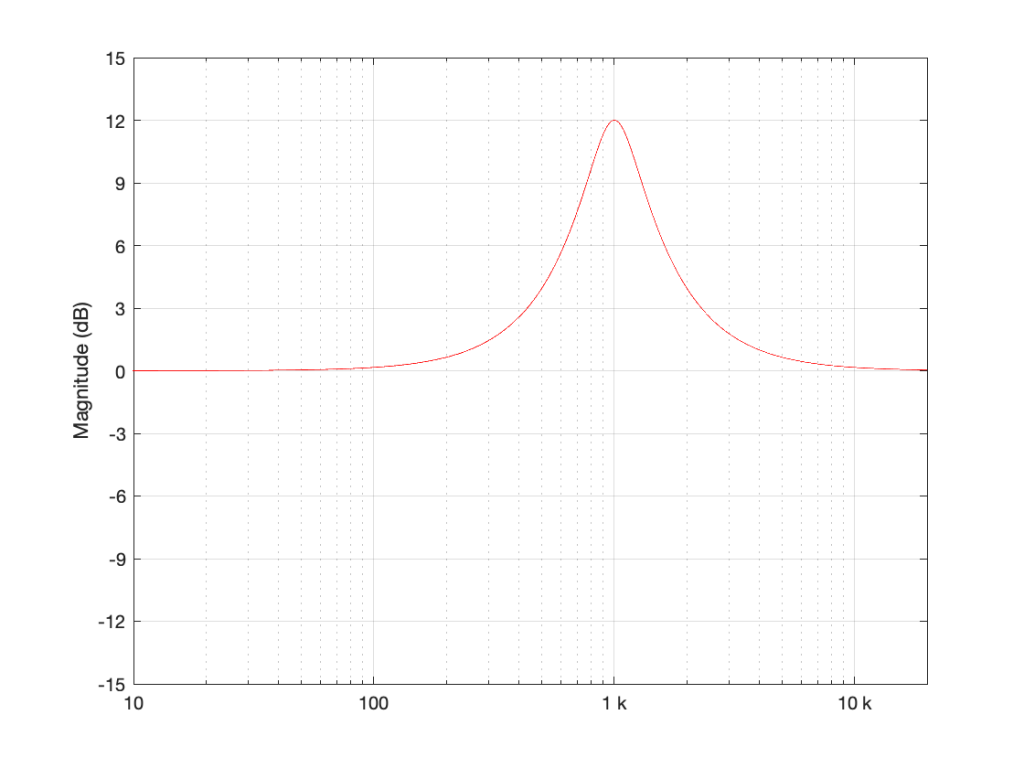Figure 1: Magnitude response of a filter with Fc=1 kHz, Gain = 12 dB, and Q=1

What happens if we implement this filter with a sampling rate of 48 kHz and 192 kHz, and then look at the difference between the two? This is shown in Figure 2.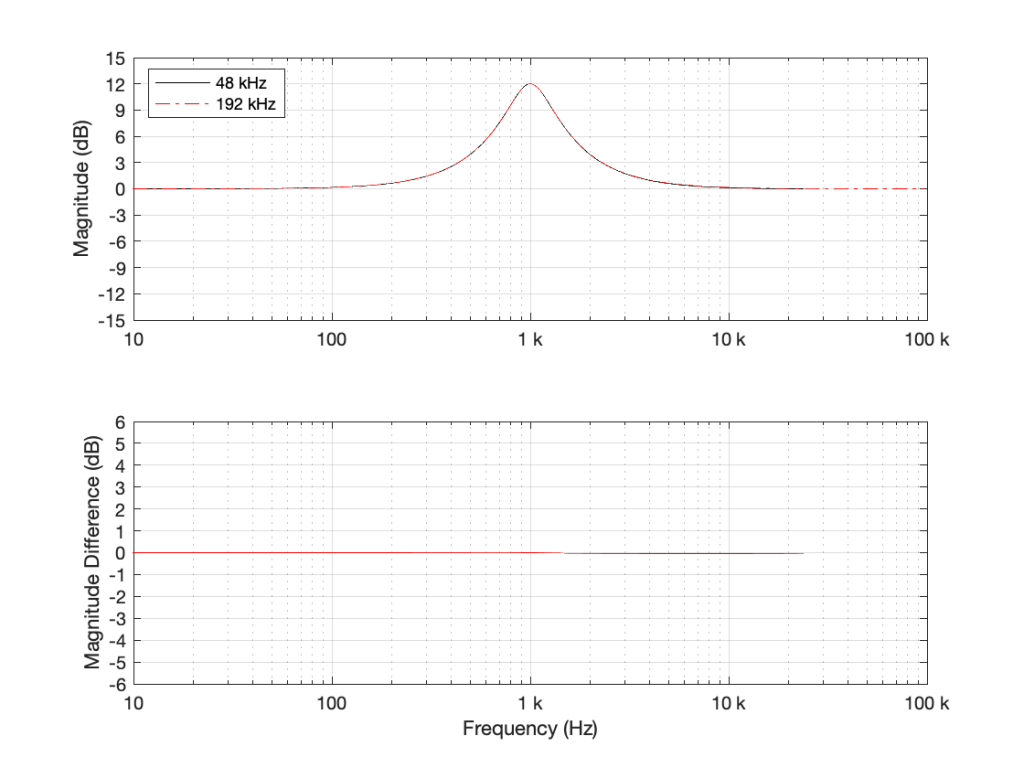Figure 2: TOP: The black curve shows the same filter from Figure 1, implemented using a biquad running at 48 kHz. The red dotted line shows the same filter, implemented using a biquad running at 192 kHz. BOTTOM: the difference between the two up to the Nyquist frequency of the 48 kHz system. (there’s almost no difference)

As you can see in Figure 2, the filter, centred at 1 kHz, is almost identical when running at 48 kHz and 192 kHz. So far so good. Now, let’s move Fc up to 10 kHz, as shown in Figure 3.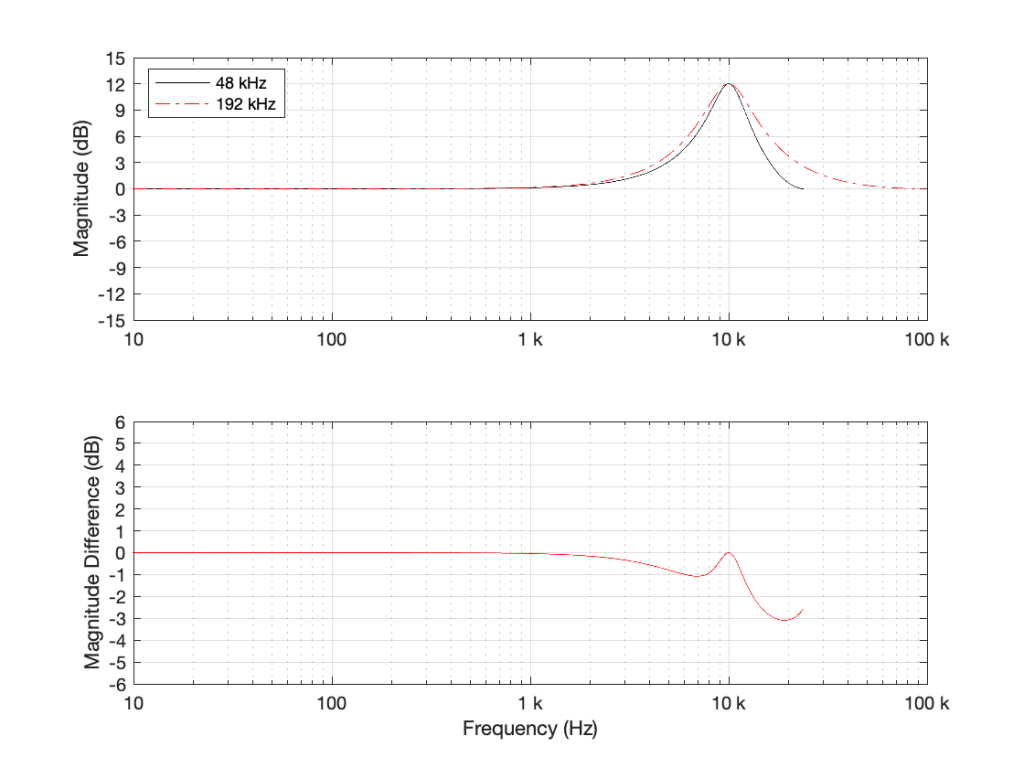Figure 3: TOP: The black curve shows the magnitude response of a filter with Fc=10 kHz, Gain = 12 dB, and Q=1, implemented using a biquad running at 48 kHz. The red dotted line shows the same filter, implemented using a biquad running at 192 kHz. BOTTOM: the difference between the two up to the Nyquist frequency of the 48 kHz system.

Take a look at the black plot on the top of Figure 3. As you can see there, the 48 kHz filter has a gain of 0 dB at 24 kHz – the Nyquist frequency. Looking at the red dotted line, we can see that the actual magnitude of the filter should have been more than +3 dB. Also, looking at the red line in the bottom plot, which shows the difference between the two curves, the 48 kHz filter starts deviating from the expected magnitude down around 1 kHz already.

So, if you want to implement a filter that behaves as you expect in the high frequency region, you’ll get better results easier with a higher sampling rate.

However, do not jump to the conclusion that this also means that you can’t implement a boost in high frequencies. For example, take a look at Figure 4, which shows a high shelving filter where Fc = 1 kHz, Gain = 12 dB and Q = 0.707.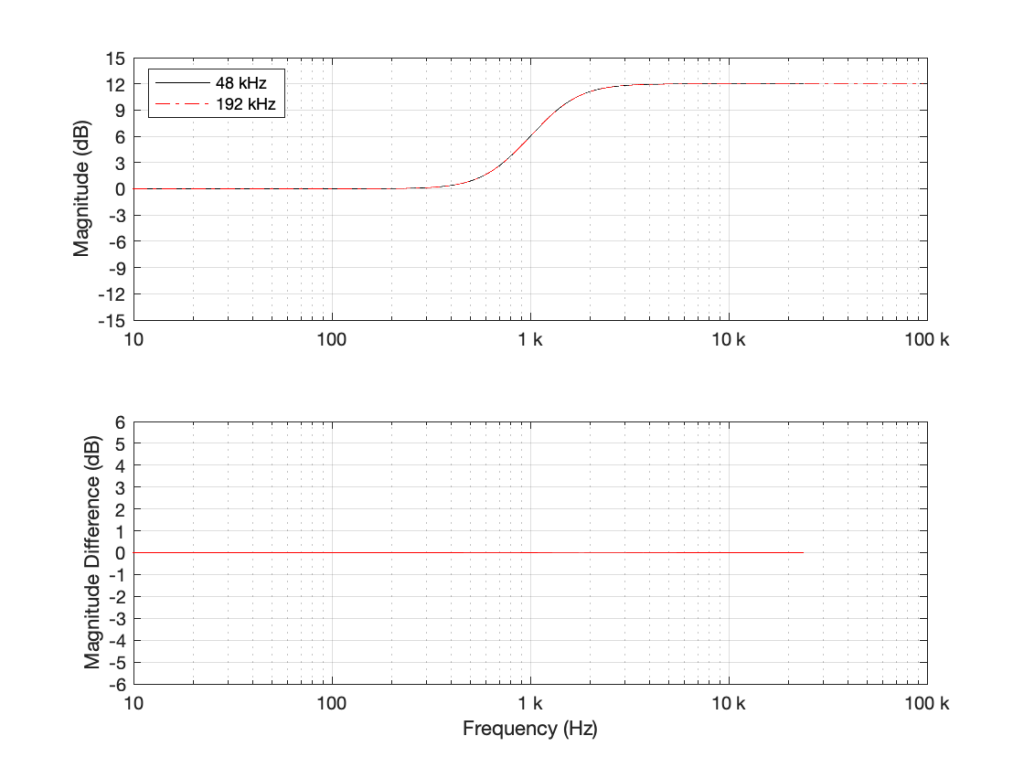Figure 4: High shelving filter where Fc = 1 kHz, Gain = 12 dB and Q = 0.707.

As you can see in the bottom plot in Figure 4, the two filters in this case (one running at 48 kHz and the other at 192 kHz) have almost identical magnitude responses. (Actually, there is a small difference of about 0.013 dB…) However, if the Fc of the shelving filter moves to 10 kHz instead (keeping the other two parameters the same) then things do get different.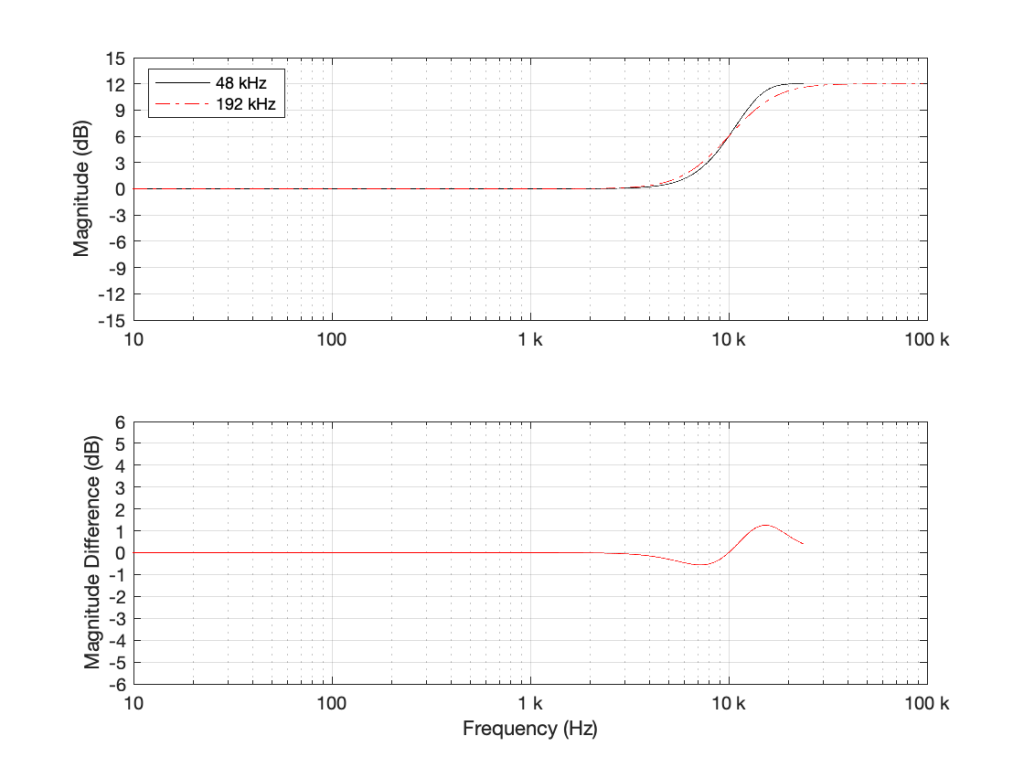Figure 5: High shelving filter where Fc = 10 kHz, Gain = 12 dB and Q = 0.707.

As can be seen there, there is a little over a 1 dB difference in the two implementations of the same filter.